# B.Tech. (SEM-I) THEORY EXAMINATION 2017-18 COMPUTER SYSTEM & PROGRAMMING IN C

Time: 3hrs
Total Marks: 100

Note:-

• There are three sections. Section A carries 20 marks, Section B carries 30 marks and Section C carries 50 marks.
• Attempt all questions. Marks are indicated against each question.
• Assume suitable data wherever necessary.

# Section – C

### 3. Attempt any two of the following: (2*5 = 10)

1. What is meant by Storage Classes of a variable? Define all types of storage classes with example.
Storage Classes are used to describe about the features of a variable/function. These features basically include the scope, visibility and life-time which help us to trace the existence of a particular variable during the runtime of a program.

C language uses 4 storage classes, namely: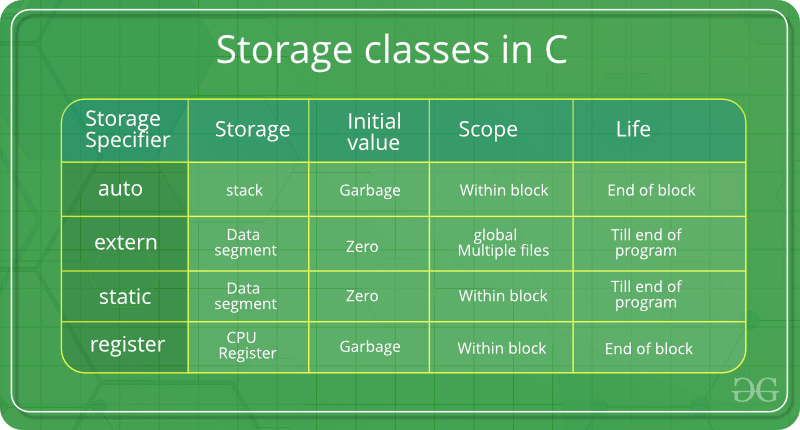1. auto: This is the default storage class for all the variables declared inside a function or a block. Hence, the keyword auto is rarely used while writing programs in C language. Auto variables can be only accessed within the block/function they have been declared and not outside them (which defines their scope). Of course, these can be accessed within nested blocks within the parent block/function in which the auto variable was declared. However, they can be accessed outside their scope as well using the concept of pointers given here by pointing to the very exact memory location where the variables resides. They are assigned a garbage value by default whenever they are declared.
2. extern: Extern storage class simply tells us that the variable is defined elsewhere and not within the same block where it is used. Basically, the value is assigned to it in a different block and this can be overwritten/changed in a different block as well. So an extern variable is nothing but a global variable initialized with a legal value where it is declared in order to be used elsewhere. It can be accessed within any function/block. Also, a normal global variable can be made extern as well by placing the ‘extern’ keyword before its declaration/definition in any function/block. This basically signifies that we are not initializing a new variable but instead we are using/accessing the global variable only. The main purpose of using extern variables is that they can be accessed between two different files which are part of a large program. For more information on how extern variables work, have a look at this link.
3. static: This storage class is used to declare static variables which are popularly used while writing programs in C language. Static variables have a property of preserving their value even after they are out of their scope! Hence, static variables preserve the value of their last use in their scope. So we can say that they are initialized only once and exist till the termination of the program. Thus, no new memory is allocated because they are not re-declared. Their scope is local to the function to which they were defined. Global static variables can be accessed anywhere in the program. By default, they are assigned the value 0 by the compiler.
4. register: This storage class declares register variables which have the same functionality as that of the auto variables. The only difference is that the compiler tries to store these variables in the register of the microprocessor if a free register is available. This makes the use of register variables to be much faster than that of the variables stored in the memory during the runtime of the program. If a free register is not available, these are then stored in the memory only. Usually few variables which are to be accessed very frequently in a program are declared with the register keyword which improves the running time of the program. An important and interesting point to be noted here is that we cannot obtain the address of a register variable using pointers.

To specify the storage class for a variable, the following syntax is to be followed:

Syntax:

`storage_class var_data_type var_name; `

Functions follow the same syntax as given above for variables. Have a look at the following C example for further clarification:

 `// C program to demonstrate different storage classes ` `#include ` ` `  `// declaring the variable which is to be made extern ` `// an intial value can also be initialized to x ` `int` `x; ` ` `  `void` `autoStorageClass() ` `{ ` ` `  `    ``printf``(``"\nDemonstrating auto class\n\n"``); ` ` `  `    ``// declaring an auto variable (simply ` `    ``// writing "int a=32;" works as well) ` `    ``auto` `int` `a = 32; ` ` `  `    ``// printing the auto variable 'a' ` `    ``printf``(``"Value of the variable 'a'"` `           ``" declared as auto: %d\n"``, ` `           ``a); ` ` `  `    ``printf``(``"--------------------------------"``); ` `} ` ` `  `void` `registerStorageClass() ` `{ ` ` `  `    ``printf``(``"\nDemonstrating register class\n\n"``); ` ` `  `    ``// declaring a register variable ` `    ``register` `char` `b = ``'G'``; ` ` `  `    ``// printing the register variable 'b' ` `    ``printf``(``"Value of the variable 'b'"` `           ``" declared as register: %d\n"``, ` `           ``b); ` ` `  `    ``printf``(``"--------------------------------"``); ` `} ` ` `  `void` `externStorageClass() ` `{ ` ` `  `    ``printf``(``"\nDemonstrating extern class\n\n"``); ` ` `  `    ``// telling the compiler that the variable ` `    ``// z is an extern variable and has been ` `    ``// defined elsewhere (above the main ` `    ``// function) ` `    ``extern` `int` `x; ` ` `  `    ``// printing the extern variables 'x' ` `    ``printf``(``"Value of the variable 'x'"` `           ``" declared as extern: %d\n"``, ` `           ``x); ` ` `  `    ``// value of extern variable x modified ` `    ``x = 2; ` ` `  `    ``// printing the modified values of ` `    ``// extern variables 'x' ` `    ``printf``(``"Modified value of the variable 'x'"` `           ``" declared as extern: %d\n"``, ` `           ``x); ` ` `  `    ``printf``(``"--------------------------------"``); ` `} ` ` `  `void` `staticStorageClass() ` `{ ` `    ``int` `i = 0; ` ` `  `    ``printf``(``"\nDemonstrating static class\n\n"``); ` ` `  `    ``// using a static variable 'y' ` `    ``printf``(``"Declaring 'y' as static inside the loop.\n"` `           ``"But this declaration will occur only"` `           ``" once as 'y' is static.\n"` `           ``"If not, then everytime the value of 'y' "` `           ``"will be the declared value 5"` `           ``" as in the case of variable 'p'\n"``); ` ` `  `    ``printf``(``"\nLoop started:\n"``); ` ` `  `    ``for` `(i = 1; i < 5; i++) { ` ` `  `        ``// Declaring the static variable 'y' ` `        ``static` `int` `y = 5; ` ` `  `        ``// Declare a non-static variable 'p' ` `        ``int` `p = 10; ` ` `  `        ``// Incrementing the value of y and p by 1 ` `        ``y++; ` `        ``p++; ` ` `  `        ``// printing value of y at each iteration ` `        ``printf``(``"\nThe value of 'y', "` `               ``"declared as static, in %d "` `               ``"iteration is %d\n"``, ` `               ``i, y); ` ` `  `        ``// printing value of p at each iteration ` `        ``printf``(``"The value of non-static variable 'p', "` `               ``"in %d iteration is %d\n"``, ` `               ``i, p); ` `    ``} ` ` `  `    ``printf``(``"\nLoop ended:\n"``); ` ` `  `    ``printf``(``"--------------------------------"``); ` `} ` ` `  `int` `main() ` `{ ` ` `  `    ``printf``(``"A program to demonstrate"` `           ``" Storage Classess in C\n\n"``); ` ` `  `    ``// To demonstrate auto Storage Class ` `    ``autoStorageClass(); ` ` `  `    ``// To demonstrate register Storage Class ` `    ``registerStorageClass(); ` ` `  `    ``// To demonstrate extern Storage Class ` `    ``externStorageClass(); ` ` `  `    ``// To demonstrate static Storage Class ` `    ``staticStorageClass(); ` ` `  `    ``// exiting ` `    ``printf``(``"\n\nStorage Classess demonstrated"``); ` ` `  `    ``return` `0; ` `} ` ` `  `// This code is improved by RishabhPrabhu `

Output:

A program to demonstrate Storage Classess in C

Demonstrating auto class

Value of the variable ‘a’ declared as auto: 32
——————————–
Demonstrating register class

Value of the variable ‘b’ declared as register: 71
——————————–
Demonstrating extern class

Value of the variable ‘x’ declared as extern: 0
Modified value of the variable ‘x’ declared as extern: 2
——————————–
Demonstrating static class

Declaring ‘y’ as static inside the loop.
But this declaration will occur only once as ‘y’ is static.
If not, then everytime the value of ‘y’ will be the declared value 5 as in the case of variable ‘p’

Loop started:

The value of ‘y’, declared as static, in 1 iteration is 6
The value of non-static variable ‘p’, in 1 iteration is 11

The value of ‘y’, declared as static, in 2 iteration is 7
The value of non-static variable ‘p’, in 2 iteration is 11

The value of ‘y’, declared as static, in 3 iteration is 8
The value of non-static variable ‘p’, in 3 iteration is 11

The value of ‘y’, declared as static, in 4 iteration is 9
The value of non-static variable ‘p’, in 4 iteration is 11

Loop ended:
——————————–

Storage Classess demonstrated

2. Wrtie a program to multiply two matrices ( read size and number of element of matrices from the keyboard).

 `// C program to multiply two square matrices. ` ` `  `#include ` ` `  `const` `int` `MAX = 100; ` ` `  `// Function to print Matrix ` `void` `printMatrix(``int` `M[][MAX], ``int` `rowSize, ``int` `colSize) ` `{ ` `    ``for` `(``int` `i = 0; i < rowSize; i++) { ` `        ``for` `(``int` `j = 0; j < colSize; j++) ` `            ``printf``(``"%d "``, M[i][j]); ` ` `  `        ``printf``(``"\n"``); ` `    ``} ` `} ` ` `  `// Function to multiply two matrices A[][] and B[][] ` `void` `multiplyMatrix(``int` `row1, ``int` `col1, ``int` `A[][MAX], ` `                    ``int` `row2, ``int` `col2, ``int` `B[][MAX]) ` `{ ` `    ``int` `i, j, k; ` ` `  `    ``// Matrix to store the result ` `    ``int` `C[MAX][MAX]; ` ` `  `    ``// Check if multiplication is Possible ` `    ``if` `(row2 != col1) { ` `        ``printf``(``"Not Possible\n"``); ` `        ``return``; ` `    ``} ` ` `  `    ``// Multiply the two ` `    ``for` `(i = 0; i < row1; i++) { ` `        ``for` `(j = 0; j < col2; j++) { ` `            ``C[i][j] = 0; ` `            ``for` `(k = 0; k < row2; k++) ` `                ``C[i][j] += A[i][k] * B[k][j]; ` `        ``} ` `    ``} ` ` `  `    ``// Print the result ` `    ``printf``(``"\nResultant Matrix: \n"``); ` `    ``printMatrix(C, row1, col2); ` `} ` ` `  `// Driven Program ` `int` `main() ` `{ ` `    ``int` `row1, col1, row2, col2, i, j; ` `    ``int` `A[MAX][MAX], B[MAX][MAX]; ` ` `  `    ``// Read size of Matrix A from user ` `    ``printf``(``"Enter the number of rows of First Matrix: "``); ` `    ``scanf``(``"%d"``, &row1); ` `    ``printf``(``"%d"``, row1); ` `    ``printf``(``"\nEnter the number of columns of First Matrix: "``); ` `    ``scanf``(``"%d"``, &col1); ` `    ``printf``(``"%d"``, col1); ` ` `  `    ``// Read the elements of Matrix A from user ` `    ``printf``(``"\nEnter the elements of First Matrix: "``); ` `    ``for` `(i = 0; i < row1; i++) { ` `        ``for` `(j = 0; j < col1; j++) { ` `            ``printf``(``"\nA[%d][%d]: "``, i, j); ` `            ``scanf``(``"%d"``, &A[i][j]); ` `            ``printf``(``"%d"``, A[i][j]); ` `        ``} ` `    ``} ` ` `  `    ``// Read size of Matrix B from user ` `    ``printf``(``"\nEnter the number of rows of Second Matrix: "``); ` `    ``scanf``(``"%d"``, &row2); ` `    ``printf``(``"%d"``, row2); ` `    ``printf``(``"\nEnter the number of columns of Second Matrix: "``); ` `    ``scanf``(``"%d"``, &col2); ` `    ``printf``(``"%d"``, col2); ` ` `  `    ``// Read the elements of Matrix B from user ` `    ``printf``(``"\nEnter the elements of First Matrix: "``); ` `    ``for` `(i = 0; i < row2; i++) { ` `        ``for` `(j = 0; j < col2; j++) { ` `            ``printf``(``"\nB[%d][%d]: "``, i, j); ` `            ``scanf``(``"%d"``, &B[i][j]); ` `            ``printf``(``"%d"``, B[i][j]); ` `        ``} ` `    ``} ` ` `  `    ``// Print the Matrix A ` `    ``printf``(``"\n\nFirst Matrix: \n"``); ` `    ``printMatrix(A, row1, col1); ` ` `  `    ``// Print the Matrix B ` `    ``printf``(``"\nSecond Matrix: \n"``); ` `    ``printMatrix(B, row2, col2); ` ` `  `    ``// Find the product of the 2 matrices ` `    ``multiplyMatrix(row1, col1, A, row2, col2, B); ` ` `  `    ``return` `0; ` `} `

Output:

```Enter the number of rows of First Matrix: 2
Enter the number of columns of First Matrix: 3
Enter the elements of First Matrix:
A: 1
A: 2
A: 3
A: 4
A: 5
A: 6

Enter the number of rows of Second Matrix: 3
Enter the number of columns of Second Matrix: 2
Enter the elements of First Matrix:
B: 1
B: 2
B: 3
B: 4
B: 5
B: 6

First Matrix:
1 2 3
4 5 6

Second Matrix:
1 2
3 4
5 6

Resultant Matrix:
22 28
49 64
```
3. Discuss various data-types used in C with suitable examples.
Each variable in C has an associated data type. Each data type requires different amounts of memory and has some specific operations which can be performed over it. Let us briefly describe them one by one:

Following are the examples of some very common data types used in C:

• char: The most basic data type in C. It stores a single character and requires a single byte of memory in almost all compilers.
• int: As the name suggests, an int variable is used to store an integer.
• float: It is used to store decimal numbers (numbers with floating point value) with single precision.
• double: It is used to store decimal numbers (numbers with floating point value) with double precision.

Different data types also have different ranges upto which they can store numbers. These ranges may vary from compiler to compiler. Below is list of ranges along with the memory requirement and format specifiers on 32 bit gcc compiler.

Data Type Memory (bytes) Range Format Specifier
short int 2 -32, 768 to 32, 767 %hd
unsigned short int 2 0 to 65, 535 %hu
unsigned int 4 0 to 4, 294, 967, 295 %u
int 4 -2, 147, 483, 648 to 2, 147, 483, 647 %d
long int 4 -2, 147, 483, 648 to 2, 147, 483, 647 %ld
unsigned long int 4 0 to 4, 294, 967, 295 %lu
long long int 8 -(2^63) to (2^63)-1 %lld
unsigned long long int 8 0 to 18, 446, 744, 073, 709, 551, 615 %llu
signed char 1 -128 to 127 %c
unsigned char 1 0 to 255 %c
float 4 %f
double 8 %lf
long double 12 %Lf

We can use the sizeof() operator to check the size of a variable.

### 4. Attempt any two of the following: (2*5 = 10)

1. What is recursion? Write a recursive program to find factorial of a number.

Recursion: The process in which a function calls itself directly or indirectly is called recursion and the corresponding function is called as recursive function. Using recursive algorithm, certain problems can be solved quite easily. Examples of such problems are Towers of Hanoi (TOH), Inorder/Preorder/Postorder Tree Traversals, DFS of Graph, etc.

Base condition in recursion: In recursive program, the solution to base case is provided and solution of bigger problem is expressed in terms of smaller problems.

Program to find factorial of a number:

 `// C program to find factorial of given number ` `#include ` ` `  `// function to find factorial of given number ` `unsigned ``int` `factorial(unsigned ``int` `n) ` `{ ` ` `  `    ``// Base case ` `    ``if` `(n == 0) ` `        ``return` `1; ` ` `  `    ``// Recursively call factorial function ` `    ``return` `n * factorial(n - 1); ` `} ` ` `  `int` `main() ` `{ ` ` `  `    ``int` `num; ` ` `  `    ``// Ge the number of which ` `    ``// factorial is to be calculated ` `    ``scanf``(``"%d"``, &num); ` `    ``printf``(``"Enter the number: %d"``, num); ` ` `  `    ``// Find the factorial ` `    ``// and print the result ` `    ``printf``(``"\nFactorial of %d is %d"``, ` `           ``num, factorial(num)); ` ` `  `    ``return` `0; ` `} `

Output:

```Enter the number: 5
Factorial of 5 is 120```
2. Explain the difference between parameter passing mechanism call by value and call by reference. Which is more efficient and why?
There are different ways in which parameter data can be passed into and out of methods and functions. Let us assume that a function B() is called from another function A(). In this case A is called the “caller function” and B is called the “called function or callee function”. Also, the arguments which A sends to B are called actual arguments and the parameters of B are called formal arguments.

Terminology

• Formal Parameter : A variable and its type as they appear in the prototype of the function or method.
• Actual Parameter : The variable or expression corresponding to a formal parameter that appears in the function or method call in the calling environment.
• Modes:
• IN: Passes info from caller to calle.
• OUT: Callee writes values in caller.
• IN/OUT: Caller tells callee value of variable, which may be updated by callee.

Important methods of Parameter Passing

1. Pass By Value : This method uses in-mode semantics. Changes made to formal parameter do not get transmitted back to the caller. Any modifications to the formal parameter variable inside the called function or method affect only the separate storage location and will not be reflected in the actual parameter in the calling environment. This method is also called as call by value.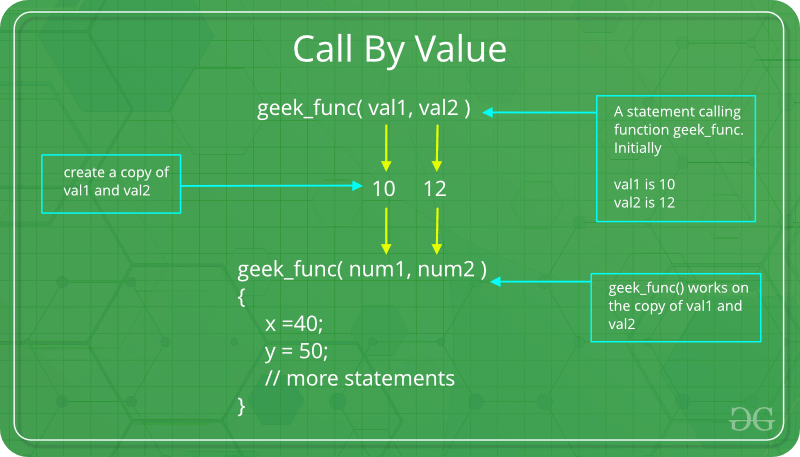`// C program to illustrate ` `// call by value ` `#include ` ` `  `void` `func(``int` `a, ``int` `b) ` `{ ` `    ``a += b; ` `    ``printf``(``"In func, a = %d b = %d\n"``, a, b); ` `} ` `int` `main(``void``) ` `{ ` `    ``int` `x = 5, y = 7; ` ` `  `    ``// Passing parameters ` `    ``func(x, y); ` `    ``printf``(``"In main, x = %d y = %d\n"``, x, y); ` `    ``return` `0; ` `} `

Output:

```In func, a = 12 b = 7
In main, x = 5 y = 7
```

Languages like C, C++, Java support this type of parameter passing. Java in fact is strictly call by value.
Shortcomings:

• Inefficiency in storage allocation
• For objects and arrays, the copy semantics are costly
2. Pass by reference(aliasing) : This technique uses in/out-mode semantics. Changes made to formal parameter do get transmitted back to the caller through parameter passing. Any changes to the formal parameter are reflected in the actual parameter in the calling environment as formal parameter receives a reference (or pointer) to the actual data. This method is also called as <em>call by reference. This method is efficient in both time and space.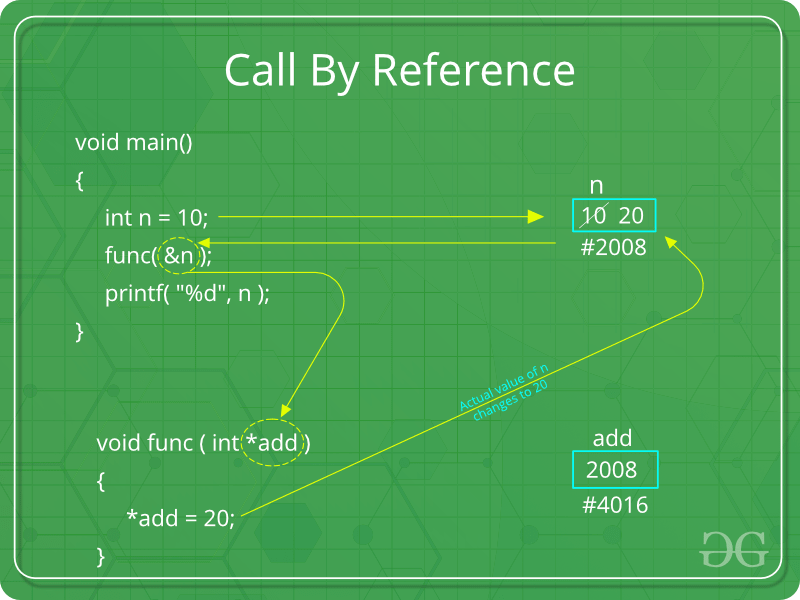`// C program to illustrate ` `// call by reference ` `#include ` ` `  `void` `swapnum(``int``* i, ``int``* j) ` `{ ` `    ``int` `temp = *i; ` `    ``*i = *j; ` `    ``*j = temp; ` `} ` ` `  `int` `main(``void``) ` `{ ` `    ``int` `a = 10, b = 20; ` ` `  `    ``// passing parameters ` `    ``swapnum(&a, &b); ` ` `  `    ``printf``(``"a is %d and b is %d\n"``, a, b); ` `    ``return` `0; ` `} `

Output:

```a is 20 and b is 10
```

C and C++ both support call by value as well as call by reference whereas Java does’nt support call by reference.
Shortcomings:

• Many potential scenarios can occur
• Programs are difficult to understand sometimes
3. Write a program to check a number is prime number or not.

Program to check a number is prime or not:

 `// C program to check if a number is prime ` ` `  `#include ` ` `  `// function check whether a number ` `// is prime or not ` `int` `isPrime(``int` `n) ` `{ ` `    ``// Corner case ` `    ``if` `(n <= 1) ` `        ``return` `0; ` ` `  `    ``// Check from 2 to n-1 ` `    ``for` `(``int` `i = 2; i < n; i++) ` `        ``if` `(n % i == 0) ` `            ``return` `0; ` ` `  `    ``return` `1; ` `} ` ` `  `// Driver Program ` `int` `main() ` `{ ` ` `  `    ``int` `num; ` ` `  `    ``// Ge the number of which ` `    ``// is to be checked ` `    ``scanf``(``"%d"``, &num); ` `    ``printf``(``"Enter the number: %d"``, num); ` ` `  `    ``// Check the number ` `    ``// and print the result ` `    ``printf``(``"\nIs %d prime: "``, num); ` ` `  `    ``if` `(isPrime(num) == 1) { ` `        ``printf``(``"Yes"``); ` `    ``} ` `    ``else` `{ ` `        ``printf``(``"No"``); ` `    ``} ` ` `  `    ``return` `0; ` `} `

Output:

```Enter the number: 5
Is 5 prime: Yes```

### 5. Attempt any two of the following: (2*5 = 10)

1. What is Macros? How it is substituted? Write macro definition with arguments for calculation of area and perimeter of a circle and rectangle. Store these macro definitions in a file called “areaperi.h”. Include this file in your program and call the macro definition for calculating area and perimeter for circle.

1. Creating areaperi.h : Write the below code and then save the file as areaperi.h. The extension should be .h indicating its a header file.

 `#define AREA_OF_CIRCLE(r) (3.14 * r * r) ` `#define PERIMETER_OF_CIRCLE(r) (3.14 * 2 * r) ` `#define AREA_OF_RECTANGLE(l, b) (l * b) ` `#define AREA_OF_RECTANGLE(l, b) (2 * (l * b)) `

2. Including the areaperi.h file in other program : Now as we need to include stdio.h as #include in order to use printf() function. We will also need to include the above header file areaperi.h as #include”areaperi.h”. The ” ” here are used to instructs the preprocessor to look into the present folder and into the standard folder of all header files if not found in present folder. So, if you wish to use angular brackets instead of ” ” to include your header file you can save it in the standard folder of header files otherwise. If you are using ” ” you need to ensure that the header file you created is saved in the same folder in which you will save the C file using this header file.
3. Using the created header file :

 `// C program to find area and perimeter ` `// of circle and rectangle ` ` `  `#include "areaperi.h" ` `#include ` ` `  `int` `main() ` `{ ` ` `  `    ``float` `radius, length, breadth; ` ` `  `    ``// Get the radius of the circle; ` `    ``scanf``(``"%f"``, &radius); ` `    ``printf``(``"Enter the radius of the circle: %f"``, radius); ` ` `  `    ``// This calls AREA_OF_CIRCLE macro ` `    ``// written in areaperi.h and ` `    ``// therefore no compilation error. ` `    ``printf``(``"\nThe area of circle: %f"``, ` `           ``AREA_OF_CIRCLE(radius)); ` ` `  `    ``// This calls PERIMETER_OF_CIRCLE macro ` `    ``// written in areaperi.h and ` `    ``// therefore no compilation error. ` `    ``printf``(``"\nThe perimeter of circle: %f"``, ` `           ``PERIMETER_OF_CIRCLE(radius)); ` ` `  `    ``// Get the length of the Rectangle ` `    ``scanf``(``"%f"``, &length); ` `    ``printf``(``"\nEnter the length of the Rectangle: %f"``, length); ` ` `  `    ``// Get the breadth of the Rectangle ` `    ``scanf``(``"%f"``, &breadth); ` `    ``printf``(``"\nEnter the breadth of the Rectangle: %f"``, breadth); ` ` `  `    ``// This calls AREA_OF_RECTANGLE macro ` `    ``// written in areaperi.h and ` `    ``// therefore no compilation error. ` `    ``printf``(``"\nThe area of Rectangle: %f"``, ` `           ``AREA_OF_RECTANGLE(length, breadth)); ` ` `  `    ``// This calls PERIMETER_OF_CIRCLE macro ` `    ``// written in areaperi.h and ` `    ``// therefore no compilation error. ` `    ``printf``(``"\nThe perimeter of Rectangle: %f"``, ` `           ``PERIMETER_OF_CIRCLE(length, breadth)); ` `} `

Output:

```
```
2. What are the different file opening modes in C? Write a program to copy the contents of one file into other file?
File opening modes in C:

• “r” – Searches file. If the file is opened successfully fopen( ) loads it into memory and sets up a pointer which points to the first character in it. If the file cannot be opened fopen( ) returns NULL.
• “w” – Searches file. If the file exists, its contents are overwritten. If the file doesn’t exist, a new file is created. Returns NULL, if unable to open file.
• “a” – Searches file. If the file is opened successfully fopen( ) loads it into memory and sets up a pointer that points to the last character in it. If the file doesn’t exist, a new file is created. Returns NULL, if unable to open file.
• “r+” – Searches file. If is opened successfully fopen( ) loads it into memory and sets up a pointer which points to the first character in it. Returns NULL, if unable to open the file.
• “w+” – Searches file. If the file exists, its contents are overwritten. If the file doesn’t exist a new file is created. Returns NULL, if unable to open file.
• “a+” – Searches file. If the file is opened successfully fopen( ) loads it into memory and sets up a pointer which points to the last character in it. If the file doesn’t exist, a new file is created. Returns NULL, if unable to open file.

C program to copy contents of one file to another file:

 `#include ` `#include // For exit() ` ` `  `int` `main() ` `{ ` `    ``FILE` `*fptr1, *fptr2; ` `    ``char` `filename, c; ` ` `  `    ``printf``(``"Enter the filename to open for reading \n"``); ` `    ``scanf``(``"%s"``, filename); ` ` `  `    ``// Open one file for reading ` `    ``fptr1 = ``fopen``(filename, ``"r"``); ` `    ``if` `(fptr1 == NULL) { ` `        ``printf``(``"Cannot open file %s \n"``, filename); ` `        ``exit``(0); ` `    ``} ` ` `  `    ``printf``(``"Enter the filename to open for writing \n"``); ` `    ``scanf``(``"%s"``, filename); ` ` `  `    ``// Open another file for writing ` `    ``fptr2 = ``fopen``(filename, ``"w"``); ` `    ``if` `(fptr2 == NULL) { ` `        ``printf``(``"Cannot open file %s \n"``, filename); ` `        ``exit``(0); ` `    ``} ` ` `  `    ``// Read contents from file ` `    ``c = ``fgetc``(fptr1); ` `    ``while` `(c != EOF) { ` `        ``fputc``(c, fptr2); ` `        ``c = ``fgetc``(fptr1); ` `    ``} ` ` `  `    ``printf``(``"\nContents copied to %s"``, filename); ` ` `  `    ``fclose``(fptr1); ` `    ``fclose``(fptr2); ` `    ``return` `0; ` `} `

Output:

```Enter the filename to open for reading
a.txt
Enter the filename to open for writing
b.txt
Contents copied to b.txt
```
3. Define structure with syntax. Also write a program that compares two given dates. To store date use structure say date that contains three members namely date, month, and year. If the dates are equal then display message as “Equal” otherwise “Unequal”.

A structure is a user-defined data type in C/C++. A structure creates a data type that can be used to group items of possibly different types into a single type.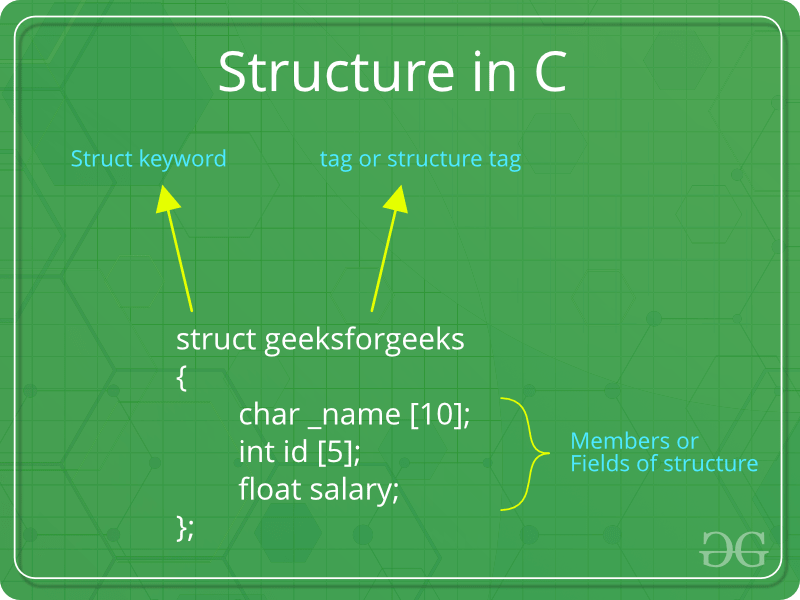How to create a structure?
‘struct’ keyword is used to create a structure. Following is an example.

 `struct` `address { ` `    ``char` `name; ` `    ``char` `street; ` `    ``char` `city; ` `    ``char` `state; ` `    ``int` `pin; ` `};`

How to declare structure variables?
A structure variable can either be declared with structure declaration or as a separate declaration like basic types.

 `// A variable declaration with structure declaration. ` `struct` `Point { ` `    ``int` `x, y; ` `} p1; ``// The variable p1 is declared with 'Point' ` ` `  `// A variable declaration like basic data types ` `struct` `Point { ` `    ``int` `x, y; ` `}; ` ` `  `int` `main() ` `{ ` `    ``struct` `Point p1; ``// The variable p1 is declared like a normal variable ` `}`

Note: In C++, the struct keyword is optional before in declaration of a variable. In C, it is mandatory.

Program that compares two given dates:

 `#include ` ` `  `// Declaring the structure of Date ` `struct` `Date { ` `    ``int` `date; ` `    ``int` `month; ` `    ``int` `year; ` `}; ` ` `  `// Driver code ` `int` `main() ` `{ ` `    ``int` `date1, date2, month1, ` `        ``month2, year1, year2; ` ` `  `    ``// Get the first date ` `    ``scanf``(``"%d"``, &date1); ` `    ``printf``(``"Enter the first date: %d"``, date1); ` `    ``scanf``(``"%d"``, &month1); ` `    ``printf``(``"\nEnter the first month: %d"``, month1); ` `    ``scanf``(``"%d"``, &year1); ` `    ``printf``(``"\nEnter the first year: %d"``, year1); ` ` `  `    ``// Initialise the structure with first date ` `    ``struct` `Date Date1 = { date1, month1, year1 }; ` ` `  `    ``// Get the second date ` `    ``scanf``(``"%d"``, &date2); ` `    ``printf``(``"\nEnter the second date: %d"``, date2); ` `    ``scanf``(``"%d"``, &month2); ` `    ``printf``(``"\nEnter the second month: %d"``, month2); ` `    ``scanf``(``"%d"``, &year2); ` `    ``printf``(``"\nEnter the second year: %d"``, year2); ` ` `  `    ``// Initialise the structure with first date ` `    ``struct` `Date Date2 = { date2, month2, year2 }; ` ` `  `    ``printf``(``"\nThe given dates are: "``); ` ` `  `    ``// Comparing the Dates ` `    ``if` `(Date1.date == Date2.date ` `        ``&& Date1.month == Date2.month ` `        ``&& Date1.year == Date2.year) { ` `        ``printf``(``"Equal"``); ` `    ``} ` `    ``else` `{ ` `        ``printf``(``"Unequal"``); ` `    ``} ` ` `  `    ``return` `0; ` `} `

Output:

```Enter the first date: 10
Enter the first month: 11
Enter the first year: 2018
Enter the second date: 10
Enter the second month: 11
Enter the second year: 2018
The given dates are: Equal
```

### 6. Attempt any two of the following: (2*5 = 10)

1. Suppose a file contains student’s records with each record containing name and age of a student. Write a C program to read these records and display them in sorted order by name.

 `// C program to read Student records  ` `// like id, name and age,  ` `// and display them in sorted order by Name  ` ` `  `#include   ` `#include   ` `#include   ` ` `  `// struct person with 3 fields  ` `struct` `Student {  ` `    ``char``* name;  ` `    ``int` `id;  ` `    ``char` `age;  ` `};  ` ` `  `// setting up rules for comparison  ` `// to sort the students based on names  ` `int` `comparator(``const` `void``* p, ``const` `void``* q)  ` `{  ` `    ``return` `strcmp``(((``struct` `Student*)p)->name,  ` `                ``((``struct` `Student*)q)->name);  ` `}  ` ` `  `// Driver program  ` `int` `main()  ` `{  ` `    ``int` `i = 0, n = 5;  ` ` `  `    ``struct` `Student arr[n];  ` ` `  `    ``// Get the students data  ` `    ``arr.id = 1;  ` `    ``arr.name = ``"bd"``;  ` `    ``arr.age = 12;  ` ` `  `    ``arr.id = 2;  ` `    ``arr.name = ``"ba"``;  ` `    ``arr.age = 10;  ` ` `  `    ``arr.id = 3;  ` `    ``arr.name = ``"bc"``;  ` `    ``arr.age = 8;  ` ` `  `    ``arr.id = 4;  ` `    ``arr.name = ``"aaz"``;  ` `    ``arr.age = 9;  ` ` `  `    ``arr.id = 5;  ` `    ``arr.name = ``"az"``;  ` `    ``arr.age = 10;  ` ` `  `    ``// Print the Unsorted Structure  ` `    ``printf``(``"Unsorted Student Records:\n"``);  ` `    ``for` `(i = 0; i < n; i++) {  ` `        ``printf``(``"Id = %d, Name = %s, Age = %d \n"``,  ` `            ``arr[i].id, arr[i].name, arr[i].age);  ` `    ``}  ` `    ``// Sort the structure  ` `    ``// based on the specified comparator  ` `    ``qsort``(arr, n, ``sizeof``(``struct` `Student), comparator);  ` ` `  `    ``// Print the Sorted Structure  ` `    ``printf``(``"\n\nStudent Records sorted by Name:\n"``);  ` `    ``for` `(i = 0; i < n; i++) {  ` `        ``printf``(``"Id = %d, Name = %s, Age = %d \n"``,  ` `            ``arr[i].id, arr[i].name, arr[i].age);  ` `    ``}  ` ` `  `    ``return` `0;  ` `}  `

Output:

```Unsorted Student Records:
Id = 1, Name = bd, Age = 12
Id = 2, Name = ba, Age = 10
Id = 3, Name = bc, Age = 8
Id = 4, Name = aaz, Age = 9
Id = 5, Name = az, Age = 10

Student Records sorted by Name:
Id = 4, Name = aaz, Age = 9
Id = 5, Name = az, Age = 10
Id = 2, Name = ba, Age = 10
Id = 3, Name = bc, Age = 8
Id = 1, Name = bd, Age = 12
```
2. Write a program to sort a set of names stored in an array in alphabetical order.

 `#include ` `#include ` `#include ` ` `  `// Defining comparator function as per the requirement ` `static` `int` `myCompare(``const` `void``* a, ``const` `void``* b) ` `{ ` ` `  `    ``// setting up rules for comparison ` `    ``return` `strcmp``(*(``const` `char``**)a, *(``const` `char``**)b); ` `} ` ` `  `// Function to sort the array ` `void` `sort(``const` `char``* arr[], ``int` `n) ` `{ ` `    ``// calling qsort function to sort the array ` `    ``// with the help of Comparator ` `    ``qsort``(arr, n, ``sizeof``(``const` `char``*), myCompare); ` `} ` ` `  `int` `main() ` `{ ` ` `  `    ``// Get the array of names to be sorted ` `    ``const` `char``* arr[]; ` `    ``= { ``"GeeksforGeeks"``, ` `        ``"GeeksQuiz"``, ` `        ``"CLanguage"` `}; ` `    ``int` `n = ``sizeof``(arr) / ``sizeof``(arr); ` `    ``int` `i; ` ` `  `    ``// Print the given names ` `    ``printf``(``"Given array of names is\n"``); ` `    ``for` `(i = 0; i < n; i++) ` `        ``printf``(``"%d: %s \n"``, i, arr[i]); ` ` `  `    ``// Sort the given names ` `    ``sort(arr, n); ` ` `  `    ``// Print the sorted names ` `    ``printf``(``"\nSorted array of names is\n"``); ` `    ``for` `(i = 0; i < n; i++) ` `        ``printf``(``"%d: %s \n"``, i, arr[i]); ` ` `  `    ``return` `0; ` `} `

Output:

```Given array of names is
0: GeeksforGeeks
1: GeeksQuiz
2: CLanguage

Sorted array of names is
0: CLanguage
1: GeeksQuiz
2: GeeksforGeeks
```
3. Write a user define function to compare two strings where they are identical or not.

 `// C program to check if ` `// two strings are identical ` ` `  `#include ` `#include ` ` `  `int` `main() ` `{ ` ` `  `    ``char` `string1, string2; ` ` `  `    ``// Get the strings which ` `    ``// is to be checked ` `    ``scanf``(``"%s"``, string1); ` `    ``printf``(``"Enter the first string: %s"``, string1); ` ` `  `    ``// Get the strings which ` `    ``// is to be checked ` `    ``scanf``(``"%s"``, string2); ` `    ``printf``(``"\nEnter the second string: %s"``, string2); ` ` `  `    ``// Check if both strings are equal ` `    ``printf``(``"\nAre both strings same: "``); ` ` `  `    ``if` `(``strcmp``(string1, string2) == 0) { ` `        ``printf``(``"Yes"``); ` `    ``} ` `    ``else` `{ ` `        ``printf``(``"No"``); ` `    ``} ` ` `  `    ``return` `0; ` `} `

Output:

```Enter the first string: GeeksForGeeks
Enter the second string: GeeksForGeeks
Are both strings same: Yes
```

### 7. Attempt any two of the following: (2*5 = 10)

1. Define the concept of pointer> Also define the dynamic memory allocation and various functions for dynamic memory allocation, with suitable examples.

Pointers are symbolic representation of addresses. They enable programs to simulate call-by-reference as well as to create and manipulate dynamic data structures. It’s general declaration in C/C++ has the format:

Syntax:

```datatype *var_name;
int *ptr;   //ptr can point to an address which holds int data
```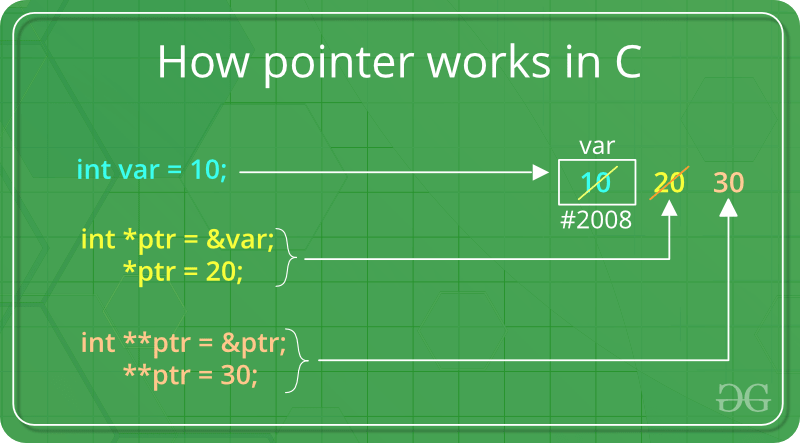How to use a pointer?

• Define a pointer variable
• Assigning the address of a variable to a pointer using unary operator (&) which returns the address of that variable.
• Accessing the value stored in the address using unary operator (*) which returns the value of the variable located at the address specified by its operand.

The reason we associate data type to a pointer is that it knows how many bytes the data is stored in. When we increment a pointer, we increase the pointer by the size of data type to which it points.Dynamic Memory Allocation in C: It can be defined as a procedure in which the size of a data structure (like Array) is changed during the runtime.

C provides some functions to achieve these tasks. There are 4 library functions provided by C defined under <stdlib.h> header file to facilitate dynamic memory allocation in C programming. They are:

1. malloc()
2. calloc()
3. free()
4. realloc()

Lets see each of them in detail.

1. malloc()

“malloc” or “memory allocation” method is used to dynamically allocate a single large block of memory with the specified size. It returns a pointer of type void which can be cast into a pointer of any form.

Syntax:

```ptr = (cast-type*) malloc(byte-size)

For Example:
ptr = (int*) malloc(100 * sizeof(int));

Since the size of int is 4 bytes,
this statement will allocate 400 bytes of memory.
And, the pointer ptr holds the address
of the first byte in the allocated memory.
```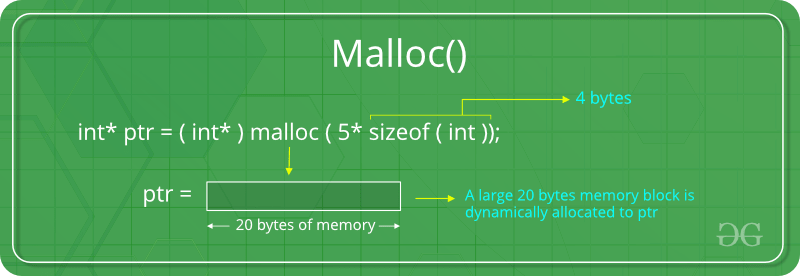If the space is insufficient, allocation fails and returns a NULL pointer.

Example:

 `#include ` `#include ` ` `  `int` `main() ` `{ ` ` `  `    ``// This pointer will hold the ` `    ``// base address of the block created ` `    ``int``* ptr; ` `    ``int` `n, i, sum = 0; ` ` `  `    ``// Get the number of elements for the array ` `    ``n = 5; ` `    ``printf``(``"Enter number of elements: %d\n"``, n); ` ` `  `    ``// Dynamically allocate memory using malloc() ` `    ``ptr = (``int``*)``malloc``(n * ``sizeof``(``int``)); ` ` `  `    ``// Check if the memory has been successfully ` `    ``// allocated by malloc or not ` `    ``if` `(ptr == NULL) { ` `        ``printf``(``"Memory not allocated.\n"``); ` `        ``exit``(0); ` `    ``} ` `    ``else` `{ ` ` `  `        ``// Memory has been successfully allocated ` `        ``printf``(``"Memory successfully allocated using malloc.\n"``); ` ` `  `        ``// Get the elements of the array ` `        ``for` `(i = 0; i < n; ++i) { ` `            ``ptr[i] = i + 1; ` `        ``} ` ` `  `        ``// Print the elements of the array ` `        ``printf``(``"The elements of the array are: "``); ` `        ``for` `(i = 0; i < n; ++i) { ` `            ``printf``(``"%d, "``, ptr[i]); ` `        ``} ` `    ``} ` ` `  `    ``return` `0; ` `} `

Output:

```Enter number of elements: 5
Memory successfully allocated using malloc.
The elements of the array are: 1, 2, 3, 4, 5,
```
2. calloc()

“calloc” or “contiguous allocation” method is used to dynamically allocate the specified number of blocks of memory of the specified type. It initializes each block with a default value ‘0’.

Syntax:

```ptr = (cast-type*)calloc(n, element-size);

For Example:
ptr = (float*) calloc(25, sizeof(float));

This statement allocates contiguous space in memory
for 25 elements each with the size of float.
```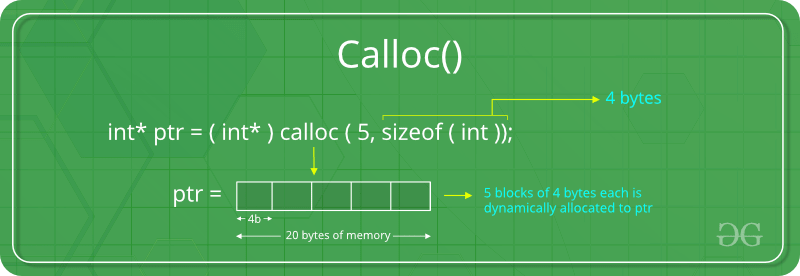If the space is insufficient, allocation fails and returns a NULL pointer.

Example:

 `#include ` `#include ` ` `  `int` `main() ` `{ ` ` `  `    ``// This pointer will hold the ` `    ``// base address of the block created ` `    ``int``* ptr; ` `    ``int` `n, i, sum = 0; ` ` `  `    ``// Get the number of elements for the array ` `    ``n = 5; ` `    ``printf``(``"Enter number of elements: %d\n"``, n); ` ` `  `    ``// Dynamically allocate memory using calloc() ` `    ``ptr = (``int``*)``calloc``(n, ``sizeof``(``int``)); ` ` `  `    ``// Check if the memory has been successfully ` `    ``// allocated by malloc or not ` `    ``if` `(ptr == NULL) { ` `        ``printf``(``"Memory not allocated.\n"``); ` `        ``exit``(0); ` `    ``} ` `    ``else` `{ ` ` `  `        ``// Memory has been successfully allocated ` `        ``printf``(``"Memory successfully allocated using calloc.\n"``); ` ` `  `        ``// Get the elements of the array ` `        ``for` `(i = 0; i < n; ++i) { ` `            ``ptr[i] = i + 1; ` `        ``} ` ` `  `        ``// Print the elements of the array ` `        ``printf``(``"The elements of the array are: "``); ` `        ``for` `(i = 0; i < n; ++i) { ` `            ``printf``(``"%d, "``, ptr[i]); ` `        ``} ` `    ``} ` ` `  `    ``return` `0; ` `} `

Output:

```Enter number of elements: 5
Memory successfully allocated using calloc.
The elements of the array are: 1, 2, 3, 4, 5,
```
3. free()

“free” method is used to dynamically de-allocate the memory. The memory allocated using functions malloc() and calloc() are not de-allocated on their own. Hence the free() method is used, whenever the dynamic memory allocation takes place. It helps to reduce wastage of memory by freeing it.

Syntax:

```free(ptr);
```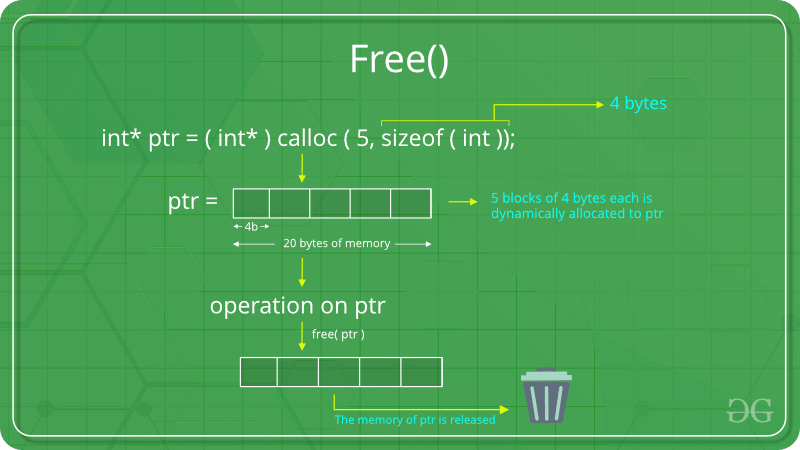Example:

 `#include ` `#include ` ` `  `int` `main() ` `{ ` ` `  `    ``// This pointer will hold the ` `    ``// base address of the block created ` `    ``int` `*ptr, *ptr1; ` `    ``int` `n, i, sum = 0; ` ` `  `    ``// Get the number of elements for the array ` `    ``n = 5; ` `    ``printf``(``"Enter number of elements: %d\n"``, n); ` ` `  `    ``// Dynamically allocate memory using malloc() ` `    ``ptr = (``int``*)``malloc``(n * ``sizeof``(``int``)); ` ` `  `    ``// Dynamically allocate memory using calloc() ` `    ``ptr1 = (``int``*)``calloc``(n, ``sizeof``(``int``)); ` ` `  `    ``// Check if the memory has been successfully ` `    ``// allocated by malloc or not ` `    ``if` `(ptr == NULL || ptr1 == NULL) { ` `        ``printf``(``"Memory not allocated.\n"``); ` `        ``exit``(0); ` `    ``} ` `    ``else` `{ ` ` `  `        ``// Memory has been successfully allocated ` `        ``printf``(``"Memory successfully allocated using malloc.\n"``); ` ` `  `        ``// Free the memory ` `        ``free``(ptr); ` `        ``printf``(``"Malloc Memory successfully freed.\n"``); ` ` `  `        ``// Memory has been successfully allocated ` `        ``printf``(``"\nMemory successfully allocated using calloc.\n"``); ` ` `  `        ``// Free the memory ` `        ``free``(ptr1); ` `        ``printf``(``"Calloc Memory successfully freed.\n"``); ` `    ``} ` ` `  `    ``return` `0; ` `} `

Output:

```Enter number of elements: 5
Memory successfully allocated using malloc.
Malloc Memory successfully freed.

Memory successfully allocated using calloc.
Calloc Memory successfully freed.
```
4. realloc()

“realloc” or “re-allocation” method is used to dynamically change the memory allocation of a previously allocated memory. In other words, if the memory previously allocated with the help of malloc or calloc is insufficient, realloc can be used to dynamically re-allocate memory.

Syntax:

```ptr = realloc(ptr, newSize);

where ptr is reallocated with new size 'newSize'.
```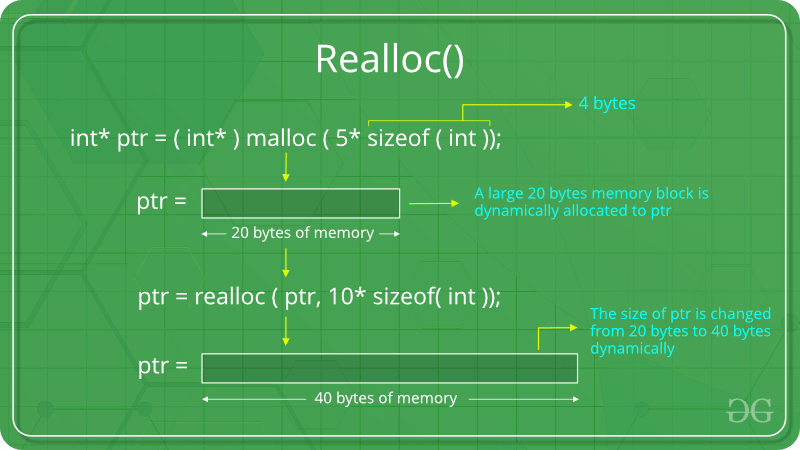If the space is insufficient, allocation fails and returns a NULL pointer.

Example:

 `#include ` `#include ` ` `  `int` `main() ` `{ ` ` `  `    ``// This pointer will hold the ` `    ``// base address of the block created ` `    ``int``* ptr; ` `    ``int` `n, i, sum = 0; ` ` `  `    ``// Get the number of elements for the array ` `    ``n = 5; ` `    ``printf``(``"Enter number of elements: %d\n"``, n); ` ` `  `    ``// Dynamically allocate memory using calloc() ` `    ``ptr = (``int``*)``calloc``(n, ``sizeof``(``int``)); ` ` `  `    ``// Check if the memory has been successfully ` `    ``// allocated by malloc or not ` `    ``if` `(ptr == NULL) { ` `        ``printf``(``"Memory not allocated.\n"``); ` `        ``exit``(0); ` `    ``} ` `    ``else` `{ ` ` `  `        ``// Memory has been successfully allocated ` `        ``printf``(``"Memory successfully allocated using calloc.\n"``); ` ` `  `        ``// Get the elements of the array ` `        ``for` `(i = 0; i < n; ++i) { ` `            ``ptr[i] = i + 1; ` `        ``} ` ` `  `        ``// Print the elements of the array ` `        ``printf``(``"The elements of the array are: "``); ` `        ``for` `(i = 0; i < n; ++i) { ` `            ``printf``(``"%d, "``, ptr[i]); ` `        ``} ` ` `  `        ``// Get the new size for the array ` `        ``n = 10; ` `        ``printf``(``"\n\nEnter the new size of the array: %d\n"``, n); ` ` `  `        ``// Dynamically re-allocate memory using realloc() ` `        ``ptr = ``realloc``(ptr, n * ``sizeof``(``int``)); ` ` `  `        ``// Memory has been successfully allocated ` `        ``printf``(``"Memory successfully re-allocated using realloc.\n"``); ` ` `  `        ``// Get the new elements of the array ` `        ``for` `(i = 5; i < n; ++i) { ` `            ``ptr[i] = i + 1; ` `        ``} ` ` `  `        ``// Print the elements of the array ` `        ``printf``(``"The elements of the array are: "``); ` `        ``for` `(i = 0; i < n; ++i) { ` `            ``printf``(``"%d, "``, ptr[i]); ` `        ``} ` ` `  `        ``free``(ptr); ` `    ``} ` ` `  `    ``return` `0; ` `} `

Output:

```Enter number of elements: 5
Memory successfully allocated using calloc.
The elements of the array are: 1, 2, 3, 4, 5,

Enter the new size of the array: 10
Memory successfully re-allocated using realloc.
The elements of the array are: 1, 2, 3, 4, 5, 6, 7, 8, 9, 10,
```
2. What is string? Also explain different string functions with examples.

Strings in C: Strings are defined as an array of characters. The difference between a character array and a string is the string is terminated with a special character ‘\0’.

Some of the most commonly used String functions are:

• strcat: The strcat() function will append a copy of the source string to the end of destination string. The strcat() function takes two arguments:
1) dest
2) src
It will append copy of the source string in the destination string. The terminating character at the end of dest is replaced by the first character of src .
Return value: The strcat() function returns dest, the pointer to the destination string.

• strchr: In C/C++, strrchr() is a predefined function used for string handling. cstring is the header file required for string functions.

This function Returns a pointer to the last occurrence of a character in a string.
The character whose last occurrence we want to find in passed as the second argument to the function and the string in which we have to find the character is passed as the first argument to the function.
Syntax

```char *strrchr(const char *str, int c)
```

Here, str is the string and c is the character to be located. It is passed as its int promotion, but it is internally converted back to char.

• strcmp: strcmp() is a built-in library function and is declared in <string.h> header file. This function takes two strings as arguments and compare these two strings lexicographically.

Syntax::

```int strcmp(const char *leftStr, const char *rightStr );
```

In the above prototype, function srtcmp takes two strings as parameters and returns an integer value based on the comparison of strings.

• strcmp() compares the two strings lexicographically means it starts comparison character by character starting from the first character until the characters in both strings are equal or a NULL character is encountered.
• If first character in both strings are equal, then this function will check the second character, if this is also equal then it will check the third and so on
• This process will be continued until a character in either string is NULL or the characters are unequal.
• strcpy: strcpy() is a standard library function in C/C++ and is used to copy one string to another. In C it is present in string.h header file and in C++ it is present in cstring header file.

Syntax:

```char* strcpy(char* dest, const char* src);
```

Paramters: This method accepts following paramters:

• dest: Pointer to the destination array where the content is to be copied.
• src: string which will be copied.

Return Value: After copying the source string to the destination string, the strcpy() function returns a pointer to the destination string.

• strlen: The strlen() function calculates the length of a given string.The strlen() function is defined in string.h header file. It doesn’t count null character ‘\0’.

Syntax:

```int strlen(const char *str);
```

Parameter:

• str: It represents the string variable whose length we have to find.

Return: This function returns the length of string passed.

• strncat: In C/C++, strncat() is a predefined function used for string handling. string.h is the header file required for string functions.

This function appends not more than n characters from the string pointed to by src to the end of the string pointed to by dest plus a terminating Null-character. The initial character of string(src) overwrites the Null-character present at the end of string(dest). Thus, length of the string(dest) becomes strlen(dest)+n. But, if the length of the string(src) is less than n, only the content up to the terminating null-character is copied and length of the string(dest) becomes strlen(src) + strlen(dest).

The behavior is undefined if

• the strings overlap.
• the dest array is not large enough to append the contents of src.

Syntax:

```char *strncat(char *dest, const char *src, size_t n)
```

Parameters: This method accepts following paramters:

• dest: the string where we want to append.
• src: the string from which ‘n’ characters are going to append.
• n: represents maximum number of character to be appended. size_t is an unsigned integral type.

Return Value: The strncat() function shall return the pointer to the string(dest).

• strncmp: std::strncmp() function lexicographically compares not more than count characters from the two null-terminated strings and returns an integer based on the outcome.
• This function takes two strings and a number num as arguments and compare at most first num bytes of both the strings.
• num should be at most equal to the length of the longest string. If num is defined greater than the string length than comparison is done till the null-character(‘\0’) of either string.
• This function compares the two strings lexicographically. It starts comparison from the first character of each string. If they are equal to each other, it continues and compare the next character of each string and so on.
• This process of comparison stops until a terminating null-character of either string is reached or num characters of both the strings matches.

Syntax :

```int strncmp(const char *str1, const char *str2, size_t count);

Parameters:
str1 and str2: C string to be compared.
count: Maximum number of characters to compare.
size_t is an unsigned integral type.

Return Value:
Value                               Meaning
Less than zero                      str1 is less than str2.
Zero                                str1 is equal to str2.
Greater than zero                   str1 is greater than str2.
```
• strncpy: The strncpy() function is similar to strcpy() function, except that at most n bytes of src are copied. If there is no NULL character among the first n character of src, the string placed in dest will not be NULL-terminated. If the length of src is less than n, strncpy() writes additional NULL character to dest to ensure that a total of n character are written.

Syntax:

`char *strncpy( char *dest, const char *src, size_t n )`

Parameters: This function accepts two parameters as mentioned above and described below:

• src: The string which will be copied.
• dest: Pointer to the destination array where the content is to be copied.
• n: The first n character copied from src to dest.

Return Value: It returns a pointer to the destination string.

• strrchr: The strrchr() function in C/C++ locates the last occurrence of a character in a string. It returns a pointer to the last occurrence in the string. The terminating null character is considered part of the C string. Therefore, it can also be located to retrieve a pointer to the end of a string. It is defined in cstring header file.
Syntax :

```const char* strrchr( const char* str, int ch )
or
char* strrchr( char* str, int ch )
```

Parameter :The function takes two mandatory parameters which are described below:

• str : specifies the pointer to the null terminated string to be searched for.
• ch: specifies the character to be search for.

Return Value: The function returns a pointer to the last location of ch in string, if the ch is found. If not found, it returns a null pointer.

3. Write short note on following (Any two):

• (i) Stack with push and pop operation: Stack is a linear data structure which follows a particular order in which the operations are performed. The order may be LIFO(Last In First Out) or FILO(First In Last Out).

Mainly the following three basic operations are performed in the stack:

• Push: Adds an item in the stack. If the stack is full, then it is said to be an Overflow condition.
• Pop: Removes an item from the stack. The items are popped in the reversed order in which they are pushed. If the stack is empty, then it is said to be an Underflow condition.
• Peek or Top: Returns top element of stack.
• isEmpty: Returns true if stack is empty, else false.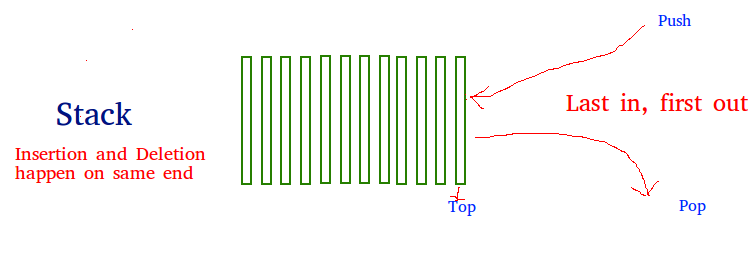How to understand a stack practically?
There are many real life examples of stack. Consider the simple example of plates stacked over one another in canteen. The plate which is at the top is the first one to be removed, i.e. the plate which has been placed at the bottommost position remains in the stack for the longest period of time. So, it can be simply seen to follow LIFO/FILO order.

Time Complexities of operations on stack:

push(), pop(), isEmpty() and peek() all take O(1) time. We do not run any loop in any of these operations.

Applications of stack:

Implementation:
There are two ways to implement a stack:

• Using array

Implementing Stack using Arrays

 `// C program for array implementation of stack ` `#include ` `#include ` `#include ` ` `  `// A structure to represent a stack ` `struct` `Stack { ` `    ``int` `top; ` `    ``unsigned capacity; ` `    ``int``* array; ` `}; ` ` `  `// function to create a stack of given capacity. It initializes size of ` `// stack as 0 ` `struct` `Stack* createStack(unsigned capacity) ` `{ ` `    ``struct` `Stack* stack = (``struct` `Stack*)``malloc``(``sizeof``(``struct` `Stack)); ` `    ``stack->capacity = capacity; ` `    ``stack->top = -1; ` `    ``stack->array = (``int``*)``malloc``(stack->capacity * ``sizeof``(``int``)); ` `    ``return` `stack; ` `} ` ` `  `// Stack is full when top is equal to the last index ` `int` `isFull(``struct` `Stack* stack) ` `{ ` `    ``return` `stack->top == stack->capacity - 1; ` `} ` ` `  `// Stack is empty when top is equal to -1 ` `int` `isEmpty(``struct` `Stack* stack) ` `{ ` `    ``return` `stack->top == -1; ` `} ` ` `  `// Function to add an item to stack.  It increases top by 1 ` `void` `push(``struct` `Stack* stack, ``int` `item) ` `{ ` `    ``if` `(isFull(stack)) ` `        ``return``; ` `    ``stack->array[++stack->top] = item; ` `    ``printf``(``"%d pushed to stack\n"``, item); ` `} ` ` `  `// Function to remove an item from stack.  It decreases top by 1 ` `int` `pop(``struct` `Stack* stack) ` `{ ` `    ``if` `(isEmpty(stack)) ` `        ``return` `INT_MIN; ` `    ``return` `stack->array[stack->top--]; ` `} ` `// Driver program to test above functions ` `int` `main() ` `{ ` `    ``struct` `Stack* stack = createStack(100); ` ` `  `    ``push(stack, 10); ` `    ``push(stack, 20); ` `    ``push(stack, 30); ` ` `  `    ``printf``(``"%d popped from stack\n"``, pop(stack)); ` ` `  `    ``return` `0; ` `} `

• Linked List: Like arrays, Linked List is a linear data structure. Unlike arrays, linked list elements are not stored at contiguous location; the elements are linked using pointers.Arrays can be used to store linear data of similar types, but arrays have following limitations.
1) The size of the arrays is fixed: So we must know the upper limit on the number of elements in advance. Also, generally, the allocated memory is equal to the upper limit irrespective of the usage.
2) Inserting a new element in an array of elements is expensive, because room has to be created for the new elements and to create room existing elements have to shifted.

For example, in a system if we maintain a sorted list of IDs in an array id[].

id[] = [1000, 1010, 1050, 2000, 2040].

And if we want to insert a new ID 1005, then to maintain the sorted order, we have to move all the elements after 1000 (excluding 1000).
Deletion is also expensive with arrays until unless some special techniques are used. For example, to delete 1010 in id[], everything after 1010 has to be moved.

1) Dynamic size
2) Ease of insertion/deletion

Drawbacks:
1) Random access is not allowed. We have to access elements sequentially starting from the first node. So we cannot do binary search with linked lists efficiently with its default implementation. Read about it here.
2) Extra memory space for a pointer is required with each element of the list.
3) Not cache friendly. Since array elements are contiguous locations, there is locality of reference which is not there in case of linked lists.

Representation:
A linked list is represented by a pointer to the first node of the linked list. The first node is called head. If the linked list is empty, then value of head is NULL.
Each node in a list consists of at least two parts:
1) data
2) Pointer (Or Reference) to the next node
In C, we can represent a node using structures. Below is an example of a linked list node with an integer data.

 `// A linked list node ` `struct` `Node { ` `    ``int` `data; ` `    ``struct` `Node* next; ` `}; `

• Command line argument: The most important function of C/C++ is main() function. It is mostly defined with a return type of int and without parameters :
```int main() { /* ... */ }
```

We can also give command-line arguments in C and C++. Command-line arguments are given after the name of the program in command-line shell of Operating Systems.
To pass command line arguments, we typically define main() with two arguments : first argument is the number of command line arguments and second is list of command-line arguments.

```int main(int argc, char *argv[]) { /* ... */ }
```

or

```int main(int argc, char **argv) { /* ... */ }
```
• argc (ARGument Count) is int and stores number of command-line arguments passed by the user including the name of the program. So if we pass a value to a program, value of argc would be 2 (one for argument and one for program name)
• The value of argc should be non negative.
• argv(ARGument Vector) is array of character pointers listing all the arguments.
• If argc is greater than zero, the array elements from argv to argv[argc-1] will contain pointers to strings.
• Argv is the name of the program, After that till argv[argc-1] every element is command -line arguments.

4. My Personal Notes arrow_drop_upCheck out this Author's contributed articles.

If you like GeeksforGeeks and would like to contribute, you can also write an article using contribute.geeksforgeeks.org or mail your article to contribute@geeksforgeeks.org. See your article appearing on the GeeksforGeeks main page and help other Geeks.

Please Improve this article if you find anything incorrect by clicking on the "Improve Article" button below.

Article Tags :

Be the First to upvote.

Please write to us at contribute@geeksforgeeks.org to report any issue with the above content.